# Subtraction of Complex Numbers

Subtraction of Complex Numbers

While doing this, sometimes, the value inside the square root may be negative.

For example, while solving a quadratic equation $$x^2+x+1=0$$ using the quadratic formula, we get: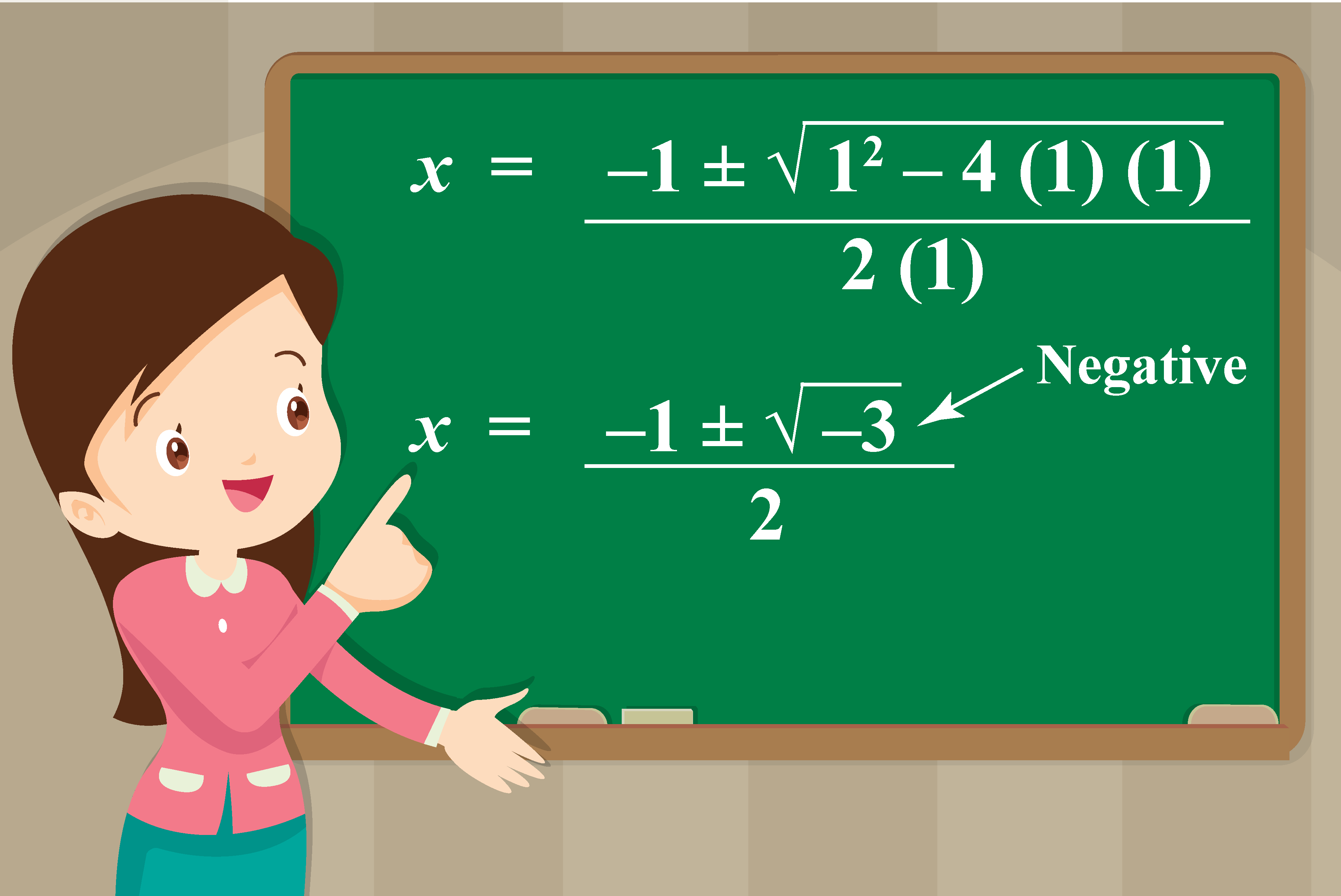So far we know that the square roots of negative numbers are NOT real numbers.

Then what type of numbers are they?

They are complex numbers

In this mini-lesson, we will learn how to subtract complex numbers and calculate complex numbers. Check-out the interactive simulations to know more about the lesson and try your hand at solving a few interesting practice questions at the end of the page.

## Lesson Plan

 1 What Do You Mean by Subtraction of Complex Numbers? 2 Important Notes on Subtraction of Complex Numbers 3 Solved Examples on Subtraction of Complex Numbers 4 Thinking Out of the Box! 5 Interactive Questions on Subtraction of Complex Numbers

## What Do You Mean by Subtraction of Complex Numbers?

We denote $$\sqrt{-1}$$ by the symbol $$i$$ which we call "iota".

So, we have $$i^2=-1$$.

This means you can say that $$i$$ is the solution of the quadratic equation $$x^2+1=0$$.

The number of the form $$z=a+ib$$, where $$a$$ and $$b$$ are real numbers are called the complex numbers.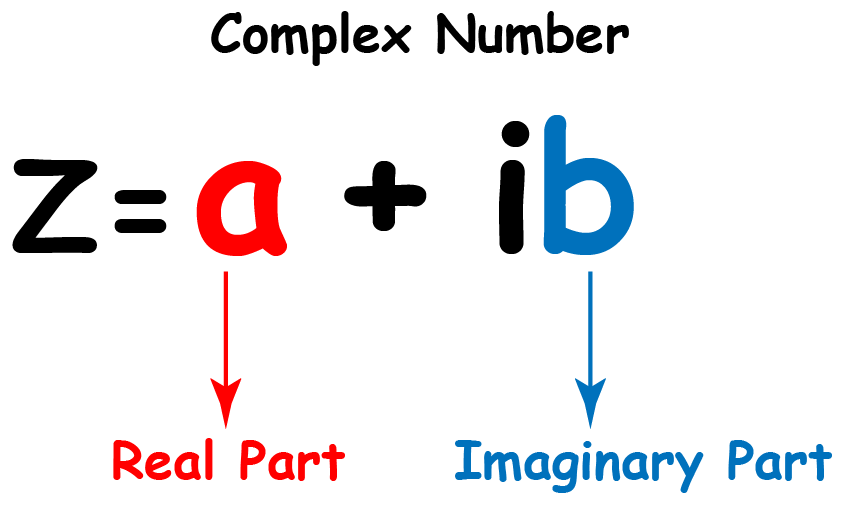Here, $$a$$ is called the real part, and $$b$$ is called the imaginary part of the complex number $$z$$.

Subtraction of complex numbers means doing the mathematical operation of subtraction on complex numbers.

### Complex Plane

Every complex number indicates a point in the XY-plane.

For example, the complex number $$x+iy$$ represents the point $$(x,y)$$ in the XY-plane.

Look at the plane shown below.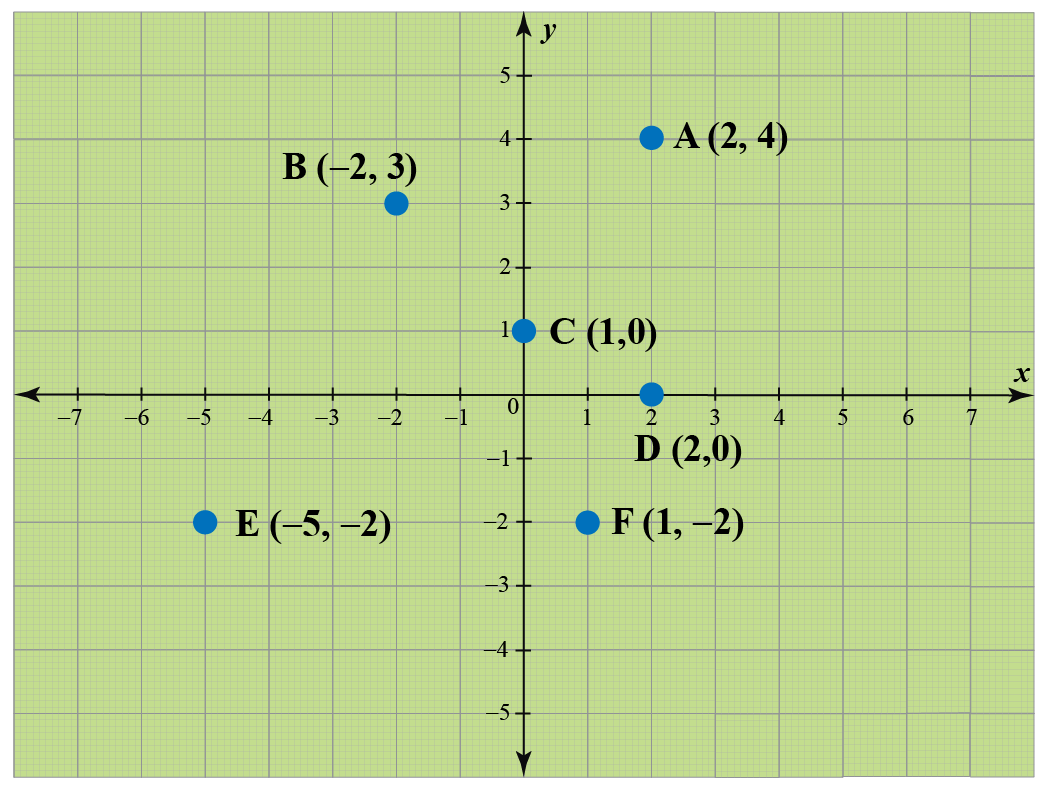The points $$(2,4), (-2,3), (0,1), (2,0), (-5,-2)$$, and $$(1,-2)$$ corresponds to the complex numbers $$2+4i, -2+3i,0+i1,2+0i,-5-2i$$, and $$1-2i$$ respectively.

The plane where a complex number is assigned to each of its points is called a complex plane.

## Parallelogram Law of Subtraction of Complex Numbers

The subtraction of complex numbers is geometrically similar to vector subtraction.

Let's see how to subtract complex numbers by using parallelogram law.

Take two complex numbers $$z_{1}=a_{1}+ib_{1}$$ and $$z_{1}=a_{1}+ib_{1}$$.

Then plot the two complex numbers in the complex plane, and consider them as normal vectors.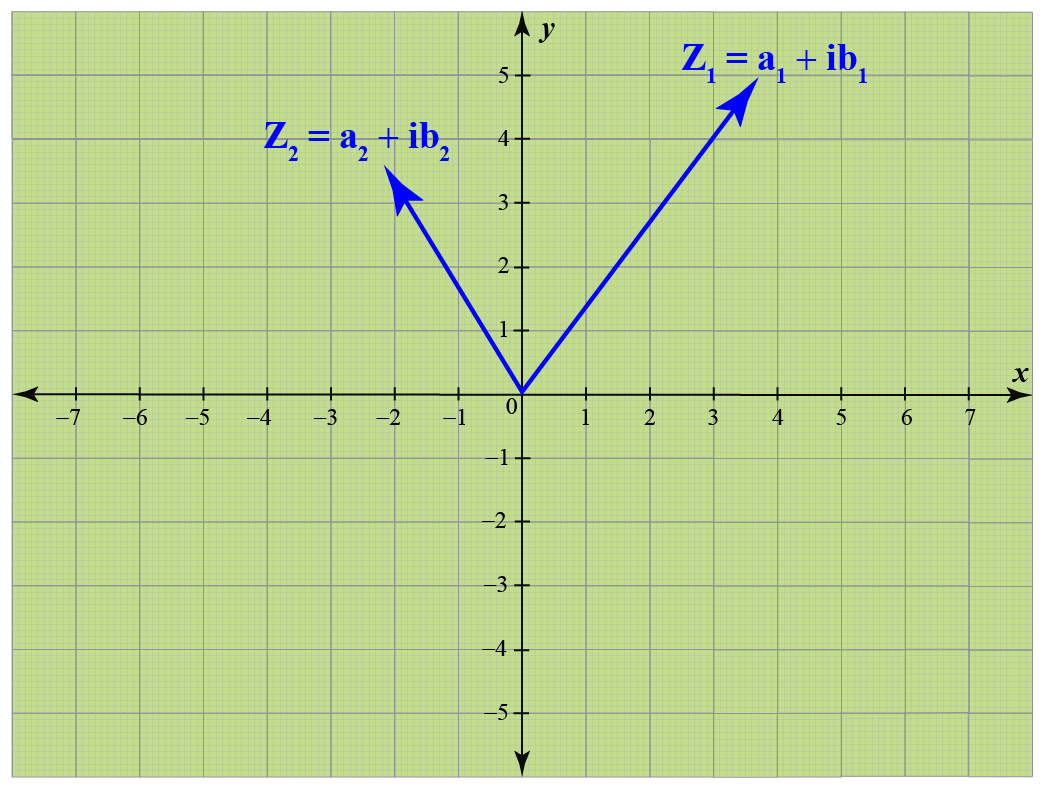Now, we need to calculate $$z_{1}-z_{2}$$.

For that we need to add the two vectors, $$z_{1}$$ and $$-z_{2}$$.

To find $$-z_{2}$$, vector reverse the $$z_{2}$$ vector with same magnitude.

Now, add the two vectors, $$z_{1}$$ and $$-z_{2}$$ by using the parallelogram law of vector addition which is shown below.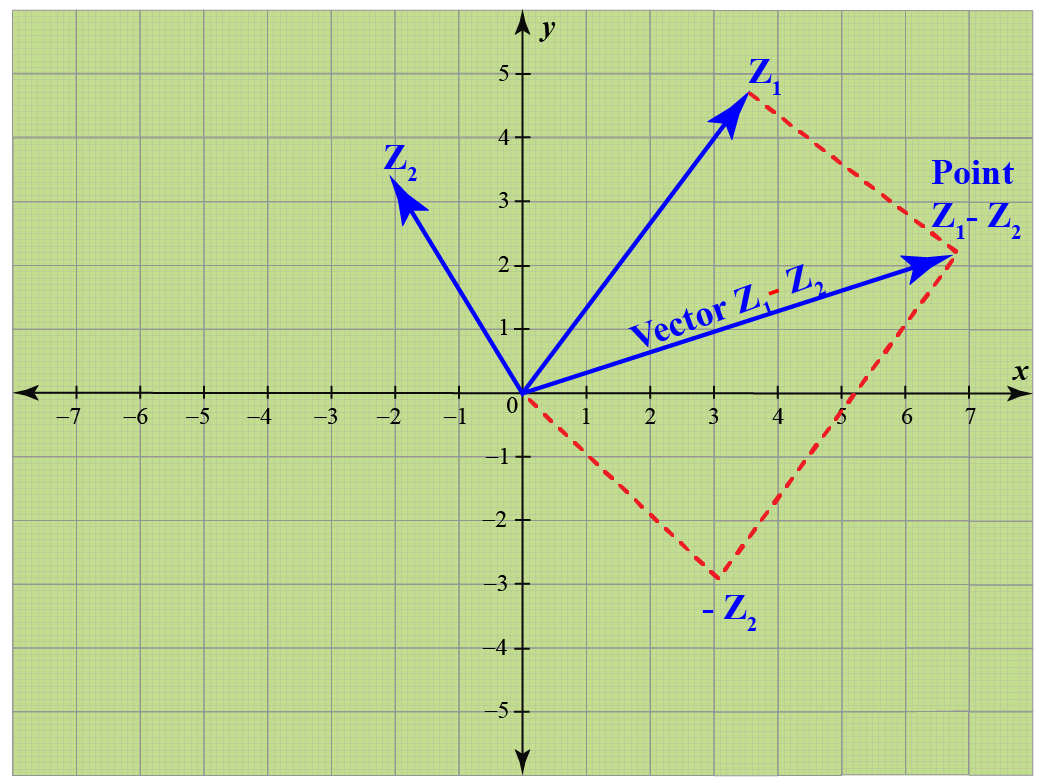The resultant vector represents the vector $$z_{1}-z_{2}$$ and its tip represent the point $$z_{1}-z_{2}$$.

Let's visualize the subtraction of complex numbers by using parallelogram law with the help of the simulation given below.

Drag the tip of the two complex vectors to visualize the parallelogram law of subtraction for different complex numbers.

## What Are the Steps to Subtract Complex Numbers?

We have already learned how to subtract complex numbers geometrically.

But isn't that process a little confusing and tough?

Let's discuss an easy process to subtract the two complex numbers.

To subtract the two complex numbers follow the steps:

1. Group the real numbers of the two complex numbers and imaginary numbers of the complex numbers.
2. Then simplify them by applying corresponding mathematical operations.

### Example

Let us subtract complex number $$z_{2}=3+4i$$ from the complex number $$z_{1}=5+7i$$ by using these steps.

\begin{aligned}z_{2}&=3+4i\\z_{1}&=5+7i\\z_{1}-z_{2}&=(5+7i)-(3+4i)\end{aligned}Now simplify by using mathematical operations:

\begin{aligned}z_{1}-z_{2}&=(5-3)+(7i-4i)\\&=2+3i\end{aligned}

Thus, the subtraction of the given two complex numbers is: $z_{1}-z_{2}= 2+3i$

Complex Numbers
grade 10 | Questions Set 1
Complex Numbers